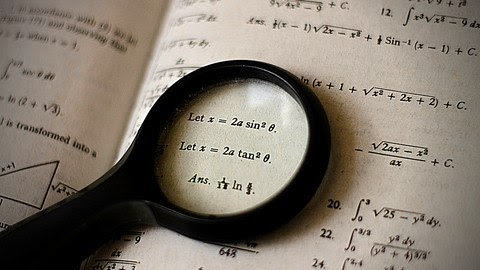July 5, 2022

# SAT Math, GRE Math, and GMAT Math: Grad School, MS, MBA Path [Free Online Course]Basic Math Foundation Skills for SAT Math, GRE Math, or GMAT Quant | Experienced Tutor with Amazing Student Testimonials

What you’ll learn

• Approach the most basic question types in the SAT, GRE, or GMAT quantitative section with confidence and deeper understanding
• Understand the basics of ratio, proportion, percentages, decimals, mixtures, rate and distance, rate and work, and rate and time
• Learn how to form and solve equations involving numbers

Description

Do you wish to learn how to approach the most basic question types tested on the SAT Math, GRE Math, or GMAT Quantitative section?  The videos cover not only the basics of but also a set of solved questions intended to make you comfortable with numbers on the SAT, GRE, or GMAT. Armed with a sound understanding of the basics, you can be assured that your dream graduate, Masters or MBA school is not far away!

When people think about standardized tests such as the SAT, GRE, or GMAT, they think expensive courses or private tutoring. The SAT, GRE, or GMAT are the path to a top graduate course, Masters program, or MBA program. No wonder they’re priced so high.

But it doesn’t have to be that way.

High fees charged by institutes aside, the glut of study material promising the moon confuses more than enlightens.

I realized: it doesn’t have to be this way.

These tests test only basic verbal and math skills, ones we’ve spent 15 years or more of our lives learning. They focus on but a fraction — pun intended — of these skills. So why not focus on study material that covers the most important concepts, and leave the rest to good, old practice?

So I explored the tests and distilled them down to the main concepts they test students on. I then created a to-the-point video course that threshes out the basics in terms of concepts, adding just enough spice via sample problems.

The result is my new course, Foundations of Math for the SAT, GRE, and GMAT.

What will YOU get from this course?

-Over 20 lectures and close to 1 hour of content!

-Understand the basics of rate, time, and distance

-Understand the basics of rate and work

-Method of solving crucial questions on rate, time, and distance

-Method of solving most frequent questions on rate and work

-Understand the basics of ratio and proportion

-Understand the basics of fractions and percentages

-Understand the basics of decimals and mixtures

-Solve critical questions on ratio and proportion

-Solve popular questions on percentages

-Solve regularly asked questions on digits, decimals, and mixtures

– Understand the basic concepts of algebra, equations, and simultaneous equations

– Understand the basic concepts of properties of lines and angles

– Understand the basic concepts of properties of triangles

– Understand the basic concepts of properties of circles

This course covers all the basics and also emphasizes how to approach problems based on the concepts. There are one or two sample questions for each topic that’s covered. I recommend that you try the sample problems on your own before looking at the solutions.

I created this course because I genuinely feel that the basics of Math tested on standardized tests such as the SAT, GRE, or GMAT are quite straightforward and should not take long hours of sifting through various resources. Of course, learning the basics is not the same as regular practice, which I highly recommend you do.

If you have any questions about any of the concepts, feel free to post in the Q and A forums. I will look at each and every question and will try to respond to each question as soon as I can. All the best.

Also See : GRE Prep & GMAT Prep Score Improvement Course

Enroll Now